# Speed of sound

(notes by Roberto Bigoni)

Sound waves are compression waves in a gas.

If a mass of gas is steady and its density ρ0 is constant throughout the occupied volume, an explosion at a point S of the volume, after some time, causes the compression of a spherical shell centered on S.

After a short time, due to the elasticity of the gas, this shell returns to its equilibrium state and expands causing compression of the adjacent outer shell, and so on, so that the spherical compression moves away from the center with constant speed v.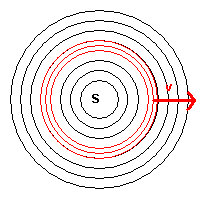To understand how this speed depends on the characteristics of the gas, we ideally isolate a beam, ie a thin straight pipe of gas, which begins on the source S and is perpendicular to all the spherical shells.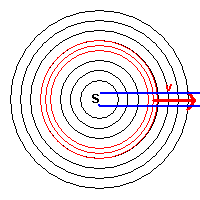In this beam, the area of compressed gas is represented by strata closer than in the unperturbed gas. If we assume that the compression propagates to the right with speed v, in a reference system fixed on the compressed zone, a point P on the right of the compressed zone moves towards the compressed zone with speed -v.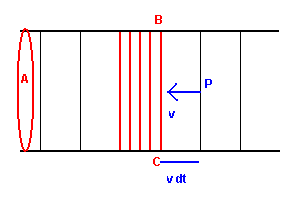If we denote by ρ0 the density of the unperturbed gas and by A the section of the beam, the infinitesimal mass dm of gas in the volume of infinitesimal thickness dx is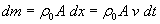On the surface BC that separates a compressed stratum from an uncompressed one, for the second law of motion, acts a force of magnitude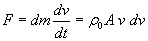Since this force is opposed to speed, its relative expression is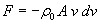This force is equal to that produced by the pressure difference dp on both sides of the surface BC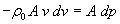We obtain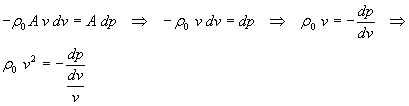Furthermore, we have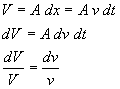and then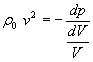The expression on the right hand side, which is the opposite of the ratio between the variation of the pressure and the relative variation of the volume, is called the bulk modulus of the gas and is usually denoted by K.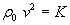In conclusion, we obtain that the speed of the compression wave in the gas compression is given by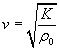Compressions and expansions of the gas strata are so fast that do not allow appreciable exchange of heat between one stratum and another and thus can be considered adiabatic processes. From the Poisson's law we have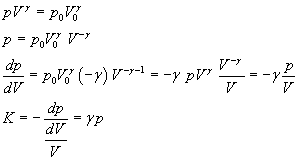therefore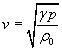Esempio

Considering the air as a diatomic ideal gas, we assume γ=1,4. At the pressure of one atmosphere (p ≅ 1,013·105Pa), the air has density ρ0 ≅ 1.225 kg/m3. The speed of sound is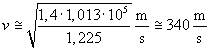Conversely, by directly measuring the speed of sound, it is possible to obtain the value of γ by an alternative method to that of Clément-Desormes: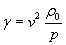Example

At the pressure of one atmosphere (p ≅ 1,013·105Pa), the air has density ρ0 ≅ 1.225 kg/m3. If a direct measurement of the speed of sound gives v = 344 m/s, then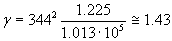last revised October 23, 2015